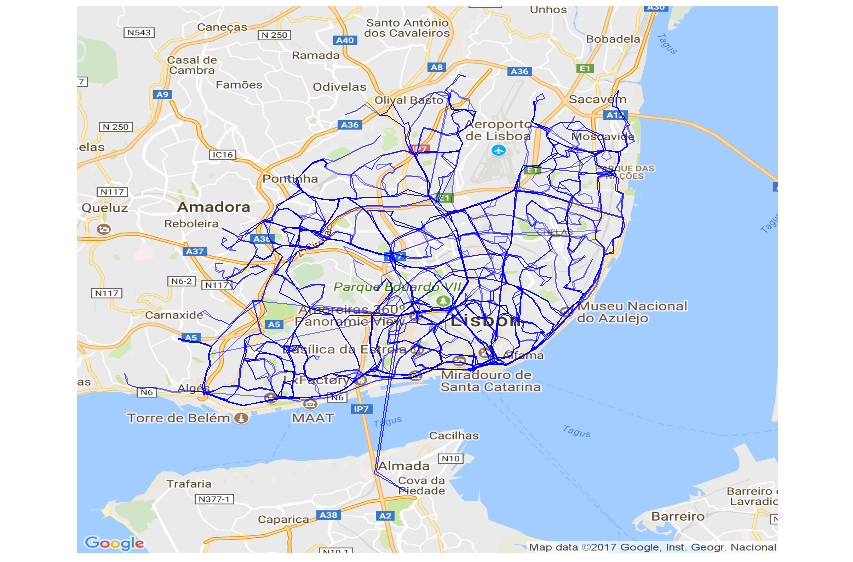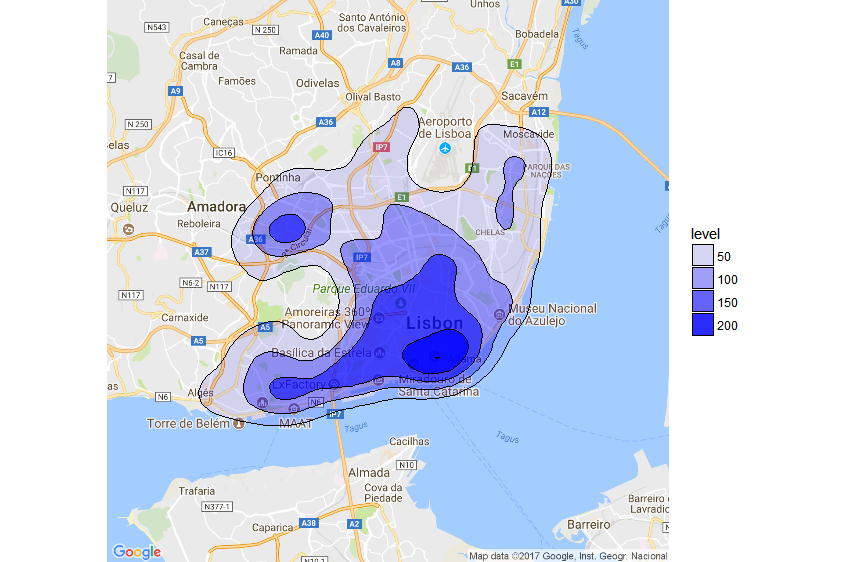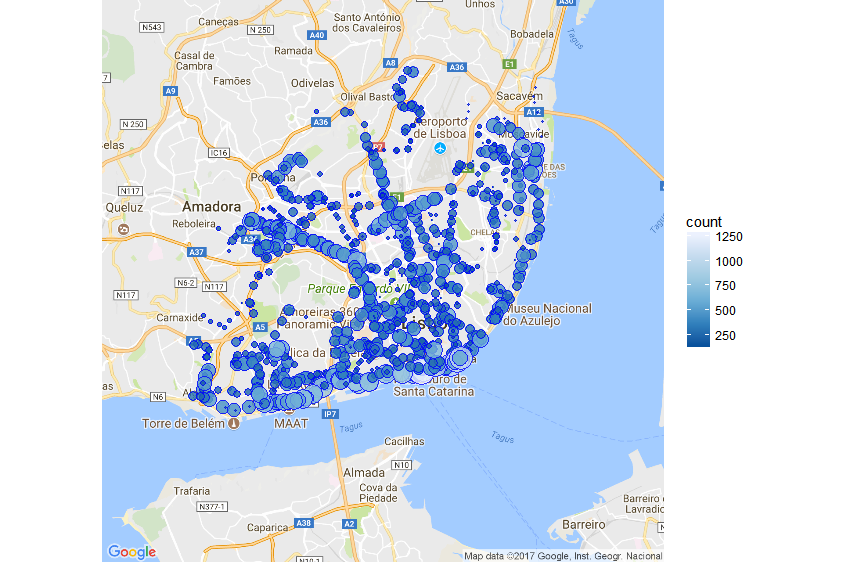{{announcement.body}}
{{announcement.title}}

# GTFS Transit Data Visualization in R

DZone 's Guide to

# GTFS Transit Data Visualization in R

### Learn how to use R with ggplot2 and ggmap to visualize GTFS (General Transit Feed Specification) route and schedule information on a map.

· Big Data Zone ·
Free Resource

Comment (2)

Save
{{ articles.views | formatCount}} Views

GTFS (General Transit Feed Specification) is a specification that defines a data format for public transportation routes, stop, schedules, and associated geographic information.

In this post, we’ll use R with ggplot2 and ggmap to visualize GTFS route and schedule information on a map.

This post uses a GTFS feed from CARRIS, which is a bus public transport operator from the city of Lisbon.

## Plot the Transport Network

Plot the whole network on a map:The code looks like this:

``````library(ggmap)
library(ggplot2)
library(ggthemes)
library(dplyr)

# fetch the map
lx_map <- get_map(location = c(-9.157513,38.73466), maptype = "roadmap", zoom = 12)
# plot the map with a line for each group of shapes (route)
ggmap(lx_map, extent = "device") +
geom_path(data = shapes, aes(shape_pt_lon, shape_pt_lat, group = shape_id), size = .1, alpha = .5, color='blue') +
coord_equal() + theme_map()``````

## Heatmap of Stops With Most Trips

Plot a heatmap of the regions with least and most number of trips. You can see in dark blue the areas with the greater number of trips.The code looks like this:

``````# read GTFS data
stop_times <- read.csv("stop_times.txt") %>% sample_n(10000) # use a data sample of 10.000 instead of the whole dataset
calendar <- read.csv("calendar.txt") %>% filterCalendar("2017-09-11") # filter trips of a given day

#join all stop times with stop info and trips
stops_freq =
inner_join(stop_times,stops,by=c("stop_id")) %>%
inner_join(trips,by=c("trip_id")) %>%
inner_join(calendar,by=c("service_id")) %>%
select(stop_id,stop_name,stop_lat,stop_lon) #%>%

# plot the map with a density/heatmap trips/stops
ggmap(lx_map, extent = "device") +
stat_density2d(data = stops_freq, aes(x = stop_lon, y = stop_lat, alpha=..level..), # variable transparency according to number of trips
size = .5, color='black', bins=5, geom = "polygon", fill='blue') # use 5 bins(transparency levels) to reprisent different densities

#################################################################
# function to filter services valid on the date filter_date_str
filterCalendar=function (calendar, filter_date_str){
calendar=calendar %>%
mutate(start_date_dt=as.Date(as.character(start_date), format="%Y%m%d")) %>%
mutate(end_date_dt  =as.Date(as.character(end_date), format="%Y%m%d"))

filter_date=as.Date(filter_date_str, format="%Y-%m-%d")
week_day=c("sunday", "monday", "tuesday", "wednesday", "thursday", "friday", "saturday")[as.POSIXlt(filter_date)\$wday + 1]
calendar[filter_date>=calendar\$start_date_dt # filter start/end dates
& filter_date<=calendar\$end_date_dt
& calendar[[week_day]] == 1 # filter the weekday
,]
}``````

## Plot Stops With Size Based on Trip Frequency

Plot a circle for each stop. The circle size and color are based on the trip frequency.The code looks like this:

``````# read GTFS stop_times

#join all data and count number of services grouped by stop
stops_freq =
inner_join(stop_times,stops,by=c("stop_id")) %>%
inner_join(trips,by=c("trip_id")) %>%
inner_join(calendar,by=c("service_id")) %>%
select(stop_id,stop_name,stop_lat,stop_lon) %>%
group_by(stop_id,stop_name,stop_lat,stop_lon) %>%
summarize(count=n()) %>%
filter(count>=150) # filter out least used stops

# plot the map with stop data
ggmap(lx_map, extent = "device") +
geom_point(data = stops_freq,aes(x=stop_lon, y=stop_lat, size=count, fill=count), shape=21, alpha=0.8, colour = "blue")+ #plot stops with blue color
scale_size_continuous(range = c(0, 9), guide = FALSE) + # size proportional to number of trips
scale_fill_distiller()  # circle fill proportional to number of trips``````

## GTFS Data Sources

Here's a list of sites where you can get GTFS feeds from multiple operators

And that's it. Enjoy!

Topics:
big data, data visualization, ggplot, r, tutorial

Comment (2)

Save
{{ articles.views | formatCount}} Views

Opinions expressed by DZone contributors are their own.